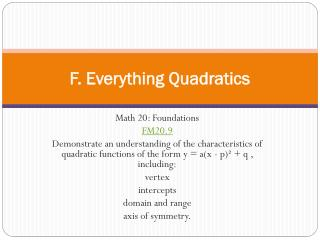DownloadDownload PresentationTélécharger la présentation- - - - - - - - - - - - - - - - - - - - - - - - - - - E N D - - - - - - - - - - - - - - - - - - - - - - - - - - -
##### Presentation Transcript

1. F. Everything Quadratics Math 20: Foundations FM20.9 Demonstrate an understanding of the characteristics of quadratic functions of the form y = a(x - p)² + q , including: vertex intercepts domain and range axis of symmetry.

2. Getting Started • String Art p.356

3. What DO YOU Think? P.357

4. 1. What is a Quadratic? • FM20.9 • Demonstrate an understanding of the characteristics of quadratic functions of the form y = a(x - p)² + q , including: • vertex • intercepts • domain and range • axis of symmetry.

5. 1. What is a Quadratic? • Quadratic Relation – A relation that can be written in the standard form, where ; for example, • Parabola – The shape of the graph of any quadratic relation.

6. Explore the Math p.359 • Grab your graphing Calculators!! • How does changing the coefficients and constant in a relation that is written in the form affect the graph of the relation?

7. Summary p.359

8. Practice • Ex. 7.1 (p.360) #1-6

9. 2. Properties of Quadratic Graphs • FM20.9 • Demonstrate an understanding of the characteristics of quadratic functions of the form y = a(x - p)² + q , including: • vertex • intercepts • domain and range • axis of symmetry.

10. Reflections p.362

11. Vertex - The point at which the quadratic function reaches its maximum or minimum value. • Axis of Symmetry - A line that separates a 2-D figure into two identical parts. For example, a parabola has a vertical axis of symmetry passing through its vertex.

12. Example 1

13. Example 2

14. Does this last Function have a max or min value?

15. Example 3

16. Summary p.368

17. Practice • Ex. 7.2 (p.368) #1-16 #4-19

18. 3. Graphing to Solve Quadratic Equations • FM20.9 • Demonstrate an understanding of the characteristics of quadratic functions of the form y = a(x - p)² + q , including: • vertex • intercepts • domain and range • axis of symmetry.

19. 3. Graphing to Solve Quadratic Equations • A zero is a number that when subbed in for the x variable it makes the equation equal to zero • A zero is another name for an x-intercept

20. Investigate the Math p.373

21. Example 1

22. Example 2

23. Example 3

24. Summary p.379

25. Practice • Ex. 6.3 (p.379) #1-13 #5-15

26. 4. Quadratics in Factored Form • FM20.9 • Demonstrate an understanding of the characteristics of quadratic functions of the form y = a(x - p)² + q , including: • vertex • intercepts • domain and range • axis of symmetry.

27. Investigate the Math p.382

28. To find your x-intercepts for your quadratic you can factor the function then set each part equal to zero and solve for x. • You can then also average your x-intercepts together to get your axis of symmetry

29. Example 1

30. Example 2

31. Example 3

32. Example 4

33. Summary p.390

34. Practice • Ex. 7.4 (p.391) #1-16 #4-20